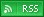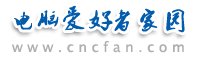电脑爱好者，提供IT资讯信息及各类编程知识文章介绍，欢迎大家来本站学习电脑知识。 最近更新 | 联系我们站内搜索：

# cmyk的4色值与rgb的3个颜色的值 如何换算

CMYK（青、品红、黄、黑）和 RGB（红、绿、蓝）是两种不同的颜色模型，它们分别用于打印和显示设备。因此，它们之间的转换不是简单的比例关系，需要进行一些复杂的计算。

R = 255 × (1 - C) × (1 - K) G = 255 × (1 - M) × (1 - K) B = 255 × (1 - Y) × (1 - K)

C = 1 - R M = 1 - G Y = 1 - B

K = min(C, M, Y)

C = (C - K) / (1 - K) × 100 M = (M - K) / (1 - K) × 100 Y = (Y - K) / (1 - K) × 100 K = K × 100

 Photoshop教学热门文章排行 网站赞助商